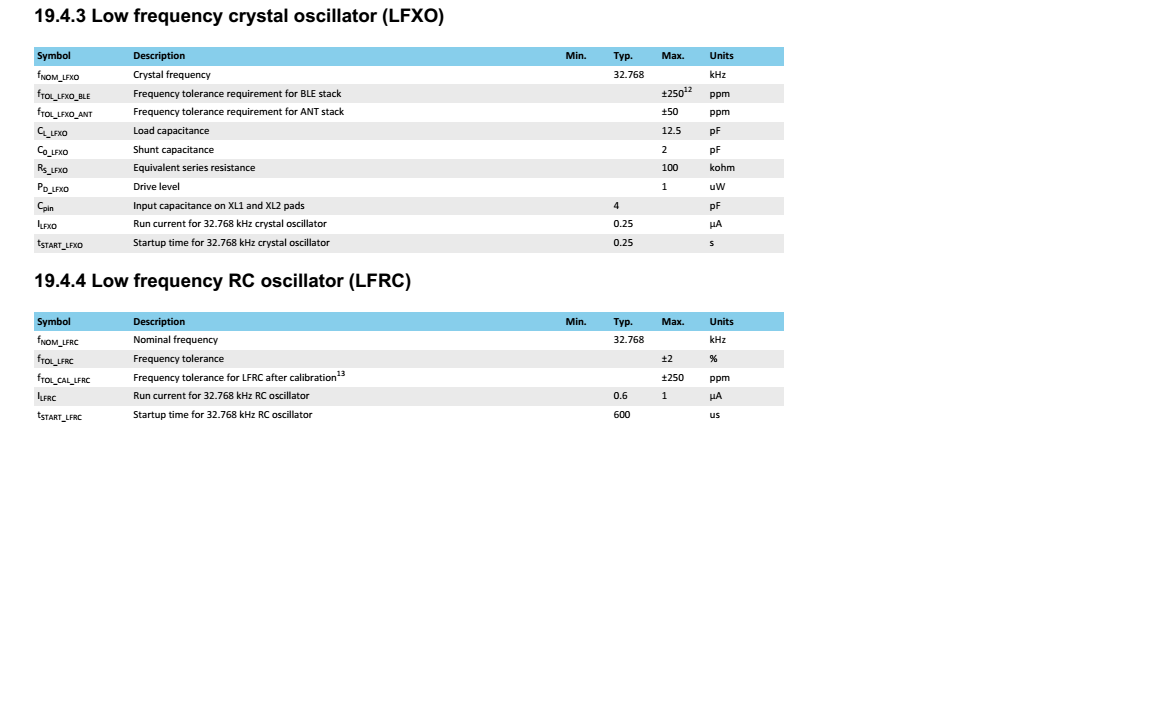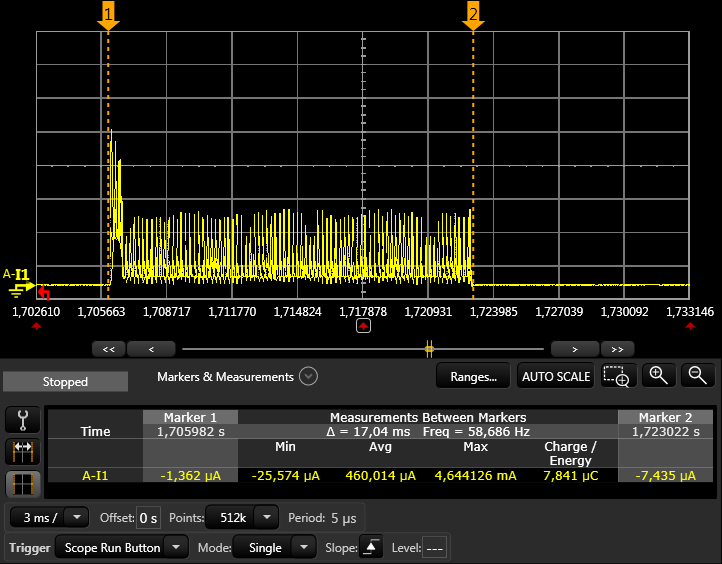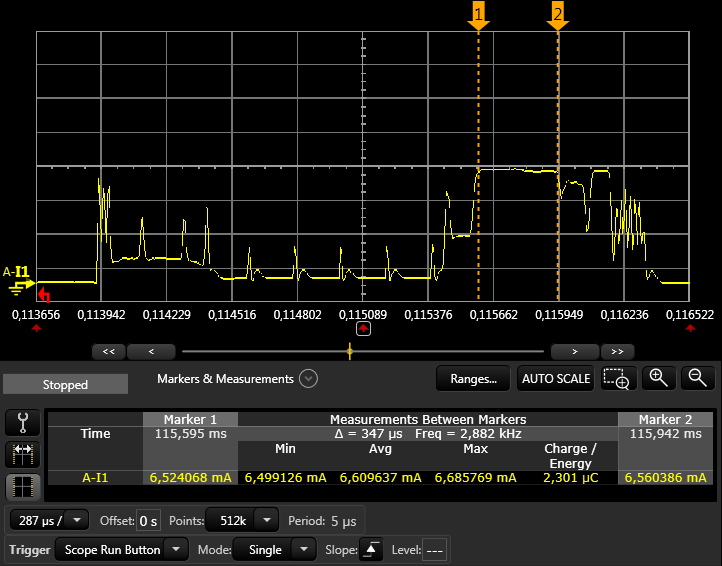# How much current consumption when we use internal 32k RC ?

I use the nRF52832 IC of WLCSP package. In addition, it sets as follows:

``````                            {.source        = NRF_CLOCK_LF_SRC_RC,            \
.rc_ctiv       = 16,                                \
.rc_temp_ctiv  = 2,                                \
.xtal_accuracy = NRF_CLOCK_LF_XTAL_ACCURACY_250_PPM}
``````

How much current consumption increases as compared with the case of using an external crystal ?

Also, please tell us in detail why the current consumption increases.

thank you

uba

• The difference in current consumption is in the product spec. Here are the tables with the information on current consumption:The reason for the power consumption increase is explained in this answer for the older nRF51822 but I imagine the reasons are similar for the newer part:

Clock power

• There are 3 factors that will affect the current consumption

1. LFRC vs LFXO run current
2. LFRC calibration
3. Window widening in connection

1. Run current

The numbers for the different run currents can be found in the product specification.

• RC: 0.6 µA
• XO: 0.25 µA

This means that the average run current will increase with 0.35µA

2. Calibration

In order to achieve a 250 ppm clock accuracy for BLE (which is required by the BLE spec), the clock must be calibrated at least every 8 seconds and every 4 seconds when the temperature changes more than 0.5 degrees (.ctiv=16, .temp_ctiv=2). See the SD spec for more info on this.

The calibration takes about 17ms and consumes 460µA in average during this time period. This results in a charge of:

``````17ms * 0.460mA = 7.82µC
``````

A calibration interval of 8 seconds gives the average current consumption:

``````7.82µC / 8s = 0.98µA
``````

Here is a plot of the calibration event:For non BLE applications the calibration might not be critical and you could use longer intervals.

3. Window widening

Window widening (will call it WW from now) is the increase of the time the RX has to listen in order to not miss the packet to receive. This is only relevant in peripheral connection mode, not in advertising mode. The peripheral listens from WW before the expected receive point in time, until WW after the expected point. This means that the time the RX is listening will increase by WW in average.

The formula to calculate the WW is as follows (from BLE core spec v4.2, Vol 6, Part B, chapter 4.5.7)

``````WW = ((masterSCA + slaveSCA)/1000000) * timeSinceLastAnchor
``````

TimeSinceLastAnchor is equal to the connection interval (no slave latency), the time since the clocks were last synced. masterSCA and slaveSCA is the sleep clock accuracy for master and slave, or if you want, the ppm accuracy you specify in code.

So if you have a 250ppm SCA (which you will have if you are using LFRC), and lets say that the central is still 20ppm, the WW will be,

``````WW = 270µ * INT
``````

where INT is the connection interval in seconds. To find the current consumed (charge) during this extra WW time, we have to multiply this with the RX current.

``````Q = WW * I_RX
``````

Now, since we want to find the average current with different connection intervals we can divide the charge (Q) with the interval, but since WW already is dependent on the interval (INT), this will cancel out and we get a constant average current we can add to all connection intervals.

``````I_ww = Q/INT
= 270µ * INT * I_RX / INT
= 270µ * I_RX
``````

The I_RX is 6.6mA (see measurements below), so then we can find the average current consumed by the WW:

``````I_ww = 270µ * 6.6mA = 1.78µA
``````

The 20ppm SCA when using LFXO will also have a small WW, and can be found in a similar way:

``````I_ww = 40µ * 6.6mA = 0.26µA (remember to add 20ppm for both master and slave = 40ppm)
``````

The increased average current when using LFRC instead of LFXO will then be,

``````1.78µA - 0.26µA = 1.52µA
``````

Here is a plot of the connection event with 250ppm slave SCA + 20ppm master SCA and a one second connection interval. We have 270µs WW and 80µs receive time = 350µs (80µs, because an empty packet consists of 80 bits, and it is transmitted at 1Mb/s)To sum up, the increased average current will be a sum of the 3 above factors

1. 0.35µA
2. 0.98µA
3. 1.52µA

Since all of the above average currents are independent of connection interval, you can add this as a constant for all connection intervals:

``````0.35 + 0.98 + 1.52 = 2.85µA
``````

And for advertising, when WW is not relevant, we get:

``````0.35 + 0.98 = 1.33µA
``````

So to finally answer your question. The expected increase in current during connection when using LFRC instead of LFXO is 2.85 µA. And during advertising a 1.33 µA increase in current.

Related